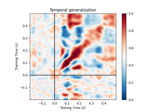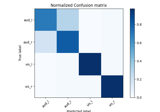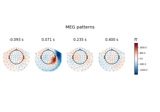# mne.decoding.Vectorizer¶

class mne.decoding.Vectorizer[source]

Transform n-dimensional array into 2D array of n_samples by n_features.

This class reshapes an n-dimensional array into an n_samples * n_features array, usable by the estimators and transformers of scikit-learn.

Examples

clf = make_pipeline(SpatialFilter(), _XdawnTransformer(), Vectorizer(),

LogisticRegression())

Attributes
features_shape_`tuple`

Stores the original shape of data.

Methods

 `__hash__`(/) Return hash(self). `fit`(X[, y]) Store the shape of the features of X. `fit_transform`(X[, y]) Fit the data, then transform in one step. Transform 2D data back to its original feature shape. Convert given array into two dimensions.
fit(X, y=None)[source]

Store the shape of the features of X.

Parameters
Xarray_like

The data to fit. Can be, for example a list, or an array of at least 2d. The first dimension must be of length n_samples, where samples are the independent samples used by the estimator (e.g. n_epochs for epoched data).

y`None` | `array`, shape (n_samples,)

Used for scikit-learn compatibility.

Returns
selfinstance of `Vectorizer`

Return the modified instance.

fit_transform(X, y=None)[source]

Fit the data, then transform in one step.

Parameters
Xarray_like

The data to fit. Can be, for example a list, or an array of at least 2d. The first dimension must be of length n_samples, where samples are the independent samples used by the estimator (e.g. n_epochs for epoched data).

y`None` | `array`, shape (n_samples,)

Used for scikit-learn compatibility.

Returns
X`array`, shape (n_samples, -1)

The transformed data.

inverse_transform(X)[source]

Transform 2D data back to its original feature shape.

Parameters
Xarray_like, shape (n_samples, n_features)

Data to be transformed back to original shape.

Returns
X`array`

The data transformed into shape as used in fit. The first dimension is of length n_samples.

transform(X)[source]

Convert given array into two dimensions.

Parameters
Xarray_like

The data to fit. Can be, for example a list, or an array of at least 2d. The first dimension must be of length n_samples, where samples are the independent samples used by the estimator (e.g. n_epochs for epoched data).

Returns
X`array`, shape (n_samples, n_features)

The transformed data.

## Examples using `mne.decoding.Vectorizer`¶